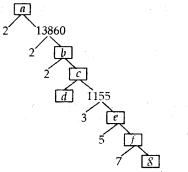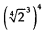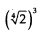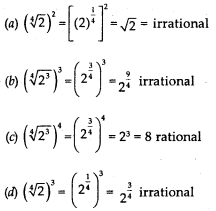# MCQ Questions for Class 10 Maths Chapter 1 Real Numbers with Answers

Students can access the NCERT MCQ Questions for Class 10 Maths Chapter 1 Real Numbers with Answers Pdf free download aids in your exam preparation and you can get a good hold of the chapter. Use MCQ Questions for Class 10 Maths with Answers during preparation and score maximum marks in the exam. Students can download the Real Numbers Class 10 MCQs Questions with Answers from here and test their problem-solving skills. Clear all the fundamentals and prepare thoroughly for the exam taking help from Class 10 Maths Chapter 1 Real Numbers Objective Questions.

## Real Numbers Class 10 MCQs Questions with Answers

Students are advised to solve the Real Numbers Multiple Choice Questions of Class 10 Maths to know different concepts. Practicing the MCQ Questions on Real Numbers Class 10 with answers will boost your confidence thereby helping you score well in the exam.

Explore numerous MCQ Questions of Real Numbers Class 10 with answers provided with detailed solutions by looking below.

Question 1.
In a seminar, the number of participants in English, German and Sanskrit are 45,75 and 135. Find the number of rooms required to house them, if in each room, the same number of participants are to be accommodated and they should be of the same language.
(a) 45
(b) 17
(c) 75
(d) 135

Since, in each room, the same number of participants, of the same language, are to be accommodated, their number in each room
HCF of 45, 75 and 135.
HCF (45, 75,135) = 15
Each room accommodates 15 participants
=> Total no. of rooms required for English = 45/15 = 3
Total no. of rooms required for German = 75/15 = 5
Total no. of rooms required for Sanskrit= 135/15 = 9
Total no. of rooms = 3 + 5 + 9 = 17

Find the Value of X calculator is a free online tool that gives the value of x when two values are given.

Question 2.
If p = HCF (100,190) and q = LCM (100, 190); then p2q2 is :
(a) 3.61 x 105
(b) 361 x 103
(c) 3.61 x 106
(d) 3.61 x 108

pq = (HCF) (LCM) = Product of given numbers.
=> pq = 190×100 =19000
=> p2q2 = 361 x 106 = 3.61 x 108

The factors of 50 obtained this way are 1, 2, 5, 10, 25, and 50.

Question 3.
A number $$\frac{p}{q}$$, when expressed in decimal form, terminates after 7 digits, then factors of q are of the form xm x yn; the value of x + y should be :
(a) 7
(b) 14
(c) 9
(d) 26

$$\frac{p}{q}$$ terminates after 8 digits
Decimal representation of $$\frac{p}{q}$$ is terminating
=> Factors of q should be of the form xm x yn
x+y=2+5=7

What is the greatest common factor of 12 and 18? Greatest Common Factor Calculator / Converter.

Question 4.
The condition to be satisfied by q, so that the rational number $$\frac{p}{q}$$ has a non terminating decimal expansion is:
(a) The prime factorisation of q is of the form 2m x 5n
(b) The prime factorisation of q is not of the form 2m x 5n
(c) The prime factorisation of q is of the form m2 x n5.
(d) The prime factorisation of q is not of the form m2 x 5n.

The prime factorisation is not of the form 2m x 5n“, where m and n are non-negative integers

Question 5.
If – 2 ≤ x ≤ 2, which of the following is not true for x:
(a) x can take only 3 values if x is a natural number.
(b) if x is an irrational number, then only one value of x is possible.
(c) if x is a non-negative integer, then only 3 values of x are possible.
(d) if x is a rational number, infinite values of x are possible.

Answer: (d) if x is a rational number, infinite values of x are possible.
– 2 ≤ x ≤ 2Question 6.
The largest positive integer which divides 434 and 539 leaving remainders 9 and 12 respectively is:
(a) 9
(b) 108
(c) 17
(d) 539

Required number is the HCF of (434 – 9] and (539 -12)
= HCF of 425 and 527.
= 17

Question 7.
There is a circular path around a field. Reema takes 22 minutes to complete one round while her friend Saina takes 20 minutes to complete the same. If they both start at the same time and move in the same direction, after how many minutes will they meet again at the starting
(a) 220
(b) 3.4
(c) 440
(d) 4.4

LCM of 20 and 22 = 220 (question state: after how many minutes will they meet)

365/838 is already in the simplest form. It can be written as 0.435561 in decimal form (rounded to 6 decimal places).

Question 8.
If HCF (306, 657) = 9, then LCM of 306 and 657 is:
(a) 1
(b) 22338
(c) 9
(d) 12

LCM (306, 657) = $$\frac{306 × 657}{9}$$

Question 9.
Euclid’s Lemma states that, for given positive integers a and b, there exist unique integers q and r, such that a = bq + r, where :
(a) 0 < r < b
(b) 0 ≤ r < b
(c) 0 < r ≤ b
(d) 0 ≤ r ≤ b

Answer: (b) 0 ≤ r < b

Question 10.
A number which can be expressed in the form $$\frac{p}{q}$$ where q ≠ 0 is a rational number is:
(a) p and q are co-prime numbers
(b) p and q are real numbers
(c) p and q are numbers
(d) p and q are integers

Answer: (d) p and q are integers

Question 11.
In decimal expansion and representation, which of the following is not a rational numbers:
(a) terminating decimal
(b) terminates after 17 digits
(c) non-terminating repeating
(d) non-terminating non-repeating

In decimal representation, a rational number is either terminating or a non- terminating but repeating.

Question 12.
If a positive integer ‘a’ is divided by 2, what can be the remainder ?
(a) 0 or 1
(b) 0,1 or 2
(c) 1 or 2
(d) Any positive number

5. When a is divided by 2, the remainder is either 0 or 1 using Euclid’s Lemma

Question 13.
For some positive integer q, every even integer is of the form:
(a) q
(b) q + 1
(c)2q
(d)2q + l

(2q + r, 0≤r≤l but 2q +1 is odd)

Question 14.
If a non-zero rational number is multiplied to an irrational number, we always get:
(a) an irrational number
(b) a rational number
(c) zero
(d) one

The product of a rational (non-zero) and m irrational number is always an irrational number.

As seen on the calculation above, we have now obtained the LCM of 15 and 20.

Question 15.
HCF and LCM of two positive integers a and b satisfy a relationship, that:
(a) (HCF)(LCM) = $$\frac{a}{b}$$
(b) (HCF) (LCM) = 1
(c) (HCF)(LCM) = ab
(d) No defined relation

7. (HCF) (LCM) = product of nos. a and b.

Question 16.
If we write 0.9 as a rational number, we get:
(a) $$\frac{9}{10}$$
(b) 1
(c) $$\frac{1}{2}$$
(d) $$\frac{1}{10}$$

8. Let x = 0.9
10x =9.9
9x = 9
x = 1

Question 17.
Write an irrational number between 2 and 3.
(a) 2.5
(b) 2.001
(c) 2.1333333456…
(d) 2.13

non terminating non repeating

Question 18.
Which of the following are irrational whose sum and product are both rationals :
(a) √2 + 3,√2 – 3
(b) √2 + √3,√2 – √3
(c) 3 + √2, 3 – √2
(d) √2 + 1,√2 – 1

Answer: (c) 3 + √2, 3 – √2
3 + √2 + 3 – √2 = 6 and (3+√2) (3-√2) =7

Question 19.
Find the value of x from the following such that x2 is irrational but x4 is rational:
(a) √2
(b) 3√2
(c) 4√2
(d) 2

√2 is a irrational number.

Question 20.
A rational number between 72 and 73 is:
(a) $$\frac{√2 + √3}{2}$$
(b) $$\frac{√2 – √3}{2}$$
(c) 1.5
(d) 1.8

Question 21.
The rational number that corresponds
to 0.6+0.$$\bar{7}$$+0.4$$\bar{7}$$ is :
(a) $$\frac{83}{90}$$
(b) $$\frac{7}{9}$$
(c) $$\frac{43}{90}$$
(d) $$\frac{167}{90}$$

Answer: (d) $$\frac{167}{90}$$
0.7 = 7/9
0.6 + 0.7 + 0.47
= 6/10 + 7/9 + 43/90
= $$\frac{167}{90}$$

Question 22.
The greatest number which divides 87 and 97, leaving 7 as remainder is :
(a) 10
(b) 1
(c) 87 x 97
(d) 6300

Greatest number which divides 87 and 97, leaving 7 as remainder = HCF of 80 and 90

Question 23.
The least number, which when divided by 10,14 and 18, leaves remainder 4, is :
a) 630
(b) 634
(c) 252
(d) 496

Required number is the LCM of 10, 14 and 18 (is 630) + 4
= 630 + 4
= 634

Question 24.
The greatest number which divides 17, 28 and 34 leaving remainders 2, 3 and 4 respectively is:
(a) 5
(b) 24
(c) 1
(d) 17

Here 17 – 2 = 15, 28 – 3 = 25, 34 – 4 = 30 Required number is the HCF of 15,25 and 30 = 5

Question 25.
72 litres of liquid A and 108 litres of liquid B are to be packed in containers of the same size. The minimum number of containers required are:
a) 36
(b) 18
(c) 5
(d) 10

For minimum number of containers required, each container should contain the maximum liquid.
Volume of each container should be the HCF of 72 and 108, which is 36. Number of containers required for liquid
A = $$\frac{72}{36}$$ = 2
Number of containers required for liquid
B = $$\frac{108}{36}$$ = 3
total = 5

Question 26.
he HCF of two consecutive rational numbers x and x +1 is :
(a) x
(b)x + 1
(c)1
(d) 0

HCF of two consecutive numbers is always 1.

Question 27.
The HCF of a number which is neither prime nor composite and any other number x is:
(a) x
(b) x + 1
(c) 1
(d) Any number

As, 1 is neither prime nor a composite number, thus, required number is the HCF of 1 and x, which is 1

Question 28.
Find q and r, if 12560 = 215 q + r.
(a) q = 58, r = 0
(b) q = 58, r = 10
(c) q = 58, r = 4
(d) q = 58, r = 90

Answer: (d) q = 58, r = 90
Using Euclid’s Lemma, 12560 when divided by 215, gives quotient as 58 and remainder as 90.
=> 12560 = 215 (58) + 90
=>q = 58 and r = 90

Question 29.
Using Euclid’s Lemma, if d is the HCF of 1155 and 506, find ‘d’
(a) 11
(b) 143
(c) 77
(d) 66

1155=506×2 + 143
506 = 143 x 3 + 77
143 =77×1 + 66
77 =66 x 1 + 11
66 =11×6 + 0
Last divisor = 11 =>
11= HCF (66,11) =
HCF (1155,506)
=>d = 11

Question 30.
An army contingent of 616 members is to march behind an army band of 32 members in a parade. The two groups have to march in the same number of columns. Find the maximum number of columns in which they can march ?
(a) 32
(b) 60
(c) 40
(d) 8

Required number is the HCF of 616 and 32 616 = 32 x 19 + 8  32 =8 x 4 + 0
HCF (616,32) = 8

Question 31.
The factor tree of a number has some unknowns, find the values of a, b, c, d, e,f and g.Answer: a = 277720, b = 6930, c = 3465, d = 3, e = 385, f = 77, g = 11

Question 32.
Which of the following is a rational number ?
(a)(b)(c)(d)Answer:Question 33.
$$\frac{91}{625}$$ ,when written in decimal form 625 terminates; as factors of denominator are in the form m2 x n5. This number will terminate after how many digits ?
(a) 3
(b) 2
(c) 1
(d) 4

= 91 x 16/104
= 1456/104 = 0.1456

Question 34.
(2 + √5)(2-√5) is:
(a) a natural number
(b) a rational number
(c) a whole number
(d) an irrational number

which is a natural number, a whole number and also a rational number.

Question 35.
Which of the following rational numbers, in decimal form, terminates ?
(a) $$\frac{64}{455}$$
(b) $$\frac{77}{210}$$
(c) $$\frac{31}{200}$$
(d) $$\frac{29}{343}$$

Answer: (c) $$\frac{31}{200}$$
(a) $$\frac{64}{455}$$
= $$\frac{64}{5 × 7 × 13}$$
= non terminating
(b) $$\frac{77}{210}$$
= $$\frac{77}{7 × 2 × 3 x 5}$$
= non terminating
(c) $$\frac{31}{200}$$
= 31/23 × 52
= terminating
(d) $$\frac{29}{343}$$
= 29/73
= non terminating

Question 36.
$$\frac{91}{625}$$ ,when written in decimal form 625 terminates; as factors of denominator are in the form m2 x n5. This number will terminate after how many digits ?
(a) 3
(b) 2
(c) 1
(d) 4

= 91 x 16/104
= 1456/104 = 0.1456

Question 37.
(2 + √5)(2-√5) is:
(a) a natural number
(b) a rational number
(c) a whole number
(d) an irrational number

which is a natural number, a whole number and also a rational number.

Question 38.
The least positive integer divisible by 20 and 24 is
(a) 360
(b) 120
(c) 480
(d) 240

Question 39.
The HCF and LCM of two numbers is 9 and 459 respectively. If one of the numbers is 27, then the other number is
(a) 459
(b) 153
(c) 135
(d) 150

Question 40.
The largest number which divides 615 and 963 leaving remainder 6 in each case is
(a) 82
(b) 95
(c) 87
(d) 93

Question 41.
The product of three consecutive integers is divisible by
(a) 5
(b) 6
(c) 7
(d) none of these

Question 42.
The least number that is divisible by all the numbers from 1 to 8 (both inclusive) is
(a) 840
(b) 2520
(c) 8
(d) 420

Question 43.
The smallest composite number is:
(a) 1
(b) 2
(c) 3
(d) 4

Question 44.
For some integer p, every odd integer is of the form
(a) 2p + 1
(b) 2p
(c) p + 1
(d) p

Question 45.
The smallest composite number is:
(a) 1
(b) 2
(c) 3
(d) 4

Question 46.
A lemma is an axiom used for proving
(a) other statement
(b) no statement
(d) none of these

Question 47.
HCF of 8, 9, 25 is
(a) 8
(b) 9
(c) 25
(d) 1

Question 48.
√7 is
(a) An integer
(b) An irrational number
(c) A rational number
(d) None of these

Question 49.
The product of a rational and irrational number is
(a) rational
(b) irrational
(c) both of above
(d) none of above

Question 50.
A number when divided by 61 gives 27 as quotient and 32 as remainder. find the number
(a) 1967
(b) 1796
(c) 1679
(d) 1569

Question 51.
For any two positive integers a and b, there exist (unique) whole numbers q and r such that
(a) q = ar + b , 0 = r < b.
(b) a = bq + r , 0 = r < b.
(c) b = aq + r , 0 = r < b.
(d) none of these

Answer: (b) a = bq + r , 0 = r < b.

Question 52.
Two natural numbers whose difference is 66 and the least common multiple is 360, are:
(a) 120 and 54
(b) 90 and 24
(c) 180 and 114
(d) 130 and 64

Question 53.
The product of a non zero rational and an irrational number is
(a) Always irrational
(b) Always rational
(c) Rational or irrational
(d) One

Question 54.
Every positive even integer is of the form ____ for some integer ‘q’.
(a) 2q
(b) 2q – 1
(c) 2q + 1
(d) none of these

Question 55.
$$\frac { 1 }{ \sqrt { 3 } }$$ is –
(a) A rational number
(b) An irrational number
(c) A whole number
(d) None of these

Question 56.
The largest number which divides 60 and 75, leaving remainders 8 and 10 respectively, is
(a) 260
(b) 75
(c) 65
(d) 13

Question 57.
p is
(a) a rational number
(b) an irrational number
(c) both (a) & (b)
(d) neither rational nor irrational

Question 58.
The largest number that will divide 398, 436 and 542 leaving remainders 7, 11 and 15 respectively is
(a) 17
(b) 11
(c) 34
(d) 45

Question 59.
Which number is divisible by 11?
(a) 1516
(b) 1452
(c) 1011
(d) 1121

Question 60.
For positive integers a and 3, there exist unique integers q and r such that a = 3q + r, where r must satisfy:
(a) 0 < r < 3
(b) 1 < r < 3
(c) 0 < r < 3
(d) 0 < r < 3

Answer: (a) 0 < r < 3

Question 61.
The decimal expansion of the rational number $$\frac { 47 }{ { 2 }^{ 3 }{ 5 }^{ 2 } }$$ will terminate after:
(a) one decimal place
(b) two decimal places
(c) three decimal places?
(d) more than three decimal places

Question 62.
The sum of a rational and an irrational number is
(a) Rational
(b) Irrational
(c) Both (a) and (c)
(d) Either (a) or (b)

Fill in the blanks:

1. Given any two positive integers a and b, there exist unique integers q and r satisfying a = bq + r and ______

2. A rational number $$\frac{p}{q}$$ will have a terminating decimal representation, if prime factors of q are of the form _____

Answer: where m and n are positive integers.

3. Rational number is a number which can be expressed in the form $$\frac{p}{q}$$, q and p, q are ______

4. If p is a prime number then √p is ________

5. Every _________ can be expressed as a product of prime numbers.

Say true or false:

Question 1.
HCF of two consecutive natural numbers n and n +1 is n.

HCF of two consecutive natural numbers is 1

Question 2.
Every composite number can be expressed as a product of primes.

Question 3.
HCF of three numbers a, b, c; when multiplied by their LCM gives the product abc

this property is true only for 2 numbers.

Question 4.
The number 6″ can never have a value which ends with the digit 0.

Question 5.
Non-terminating and Non-recurring decimals are called Irrational Numbers.

Match the following:

Question 1.

 1. 4.37$$\bar{2}$$ a. $$\frac{1093}{250}$$ 2. 4.3$$\bar{72}$$ b. $$\frac{787}{180}$$ 3. 4. $$\bar{372}$$ c. $$\frac{1456}{333}$$ 4. 4.372 d. $$\frac{4329}{990}$$

 1. 4.37$$\bar{2}$$ b. $$\frac{787}{180}$$ 2. 4.3$$\bar{72}$$ d. $$\frac{4329}{990}$$ 3. 4. $$\bar{372}$$ c. $$\frac{1456}{333}$$ 4. 4.372 a. $$\frac{1093}{250}$$

Question 2.

 a. it is the greatest positive integer which divides both the intergers. Rational number 4.37 b. it is the lowest of their common multiples. HCF Of two consecutive numbers is 1 c. terminating decimal expansion. Irrational number Of two consecutive numbers is their product. d. non terminating non repeating decimal expansion. LCM 2.101001000110000…….

 a. it is the greatest positive integer which divides both the intergers. HCF Of two consecutive numbers is 1 b. it is the lowest of their common multiples. LCM Of two consecutive numbers is their product. c. terminating decimal expansion. Rational number 4.37 d. non terminating non repeating decimal expansion. Irrational number 2.101001000110000…….

Classify the following as Rational and Irrational:

Question 1.
√9

Question 2.
2 + √2

Question 3.
$$\frac { π }{ 2 }$$

Question 4.
0.666………

Question 5.
1.7324

Question 6.
√4 + √7

Question 7.
0.7347896……..

Question 8.
4.363636……

Question 9.
– 7 – √3